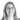In the startup world, the two most widely used methods for raising funds are through equity and convertible notes. In this post, we’ll share with you real-world data from our app and some additional insights about convertible notes. When reading about it elsewhere you may also encounter the terms debt financing, convertible debt and convertible loan.

1. What Is a Convertible Note and Why Do Startups Choose It?
2. Examples from the Real World: Typical Parameters
3. How to Add Convertible Notes in Ledgy

### 1. What Is a Convertible Note and Why Do Startups Choose It?

A convertible note is debt that is eventually either converted into equity (typically at the next equity round) or is paid back to the investor. Convertible notes can also be known as bridge investments because they bridge the company until the next equity round. Usually, the conversion from debt to equity is at a discounted price (lower when compared to the next equity round’s price). It can also have a cap, or maximum price, which protects the investor in the event that the company’s valuation becomes too high.

The convertible note can convert into equity only during an equity round. Much like regular loans, convertible loans often have an interest rate and a maturity date. A maturity date is the date in the future by which the debt should be repaid, with interest (if applicable). The maturity date is essentially the time a startup has to raise money via an equity round.

For example, let’s say a company has a convertible note with a maturity date of 18 months from the date of investment. In the event that the company hasn’t had an equity round within 18 months, the investor could request her money back. Usually, investors will renegotiate the maturity date, rather than demand repayment.

The benefits of a convertible note round, in comparison to an equity round, are speed, lower cost of execution and no need for a valuation of the company. An average convertible note contract takes just a couple of hours to prepare and costs between €2,500 and €5,000 to create.

In the last 10 years, new variations on the convertible note have been introduced. Y Combinator introduced SAFE (Simple agreement for future equity), 500 startups KISS (Keep it simple security) and the Founder Institute brought to life “convertible equity”.

Startups tend to have a hard time properly valuing their company in the seed round—the company typically doesn’t have either assets or customers. However, in order to raise money with equity financing, the value of the company has to be set. Many startups and investors find themselves in a situation where they don’t agree on the value of the company, and for that reason, they turn to convertible loans. Startups receive the money now and the valuation is decided in a later round.

### 2. Examples from the Real World: What Are Typically Used Parameters?

Before we looked at our own data, we scoured the web and found some information about typical parameters.

• The typical convertible loan ranges from €100k to a couple of million.
• Discounts range from 0% to as high as 35%. A typical discount would be 15–25%.
• The maturity date is usually set at 18–24 months after the date of the convertible note investment.
• The Interest rate is most often between 2–8%.

Data about Convertible Notes from the Ledgy App

a) Convertible note size

In our database, there’s a large number of convertible loans in the range of 0–50k and almost no loans of more than 1m.

Note: Investment amount ranges include investments between the lower number and up to the higher number: for example, the range from 0–50k includes investments above 0 including 50k.

b) Discount

Our data about discounts is comparable to what we found during our initial research online. Additionally, we can see that a little over 50% of convertible notes had a 0% discount.

c) Valuation Cap

A typical cap is hard to determine since it depends on the company’s potential value at the time the convertible is negotiated. However, we can look at the ratio of the convertible note’s amount to the cap. For example, if an investor invests €100,000 through a convertible with a cap of €1,000,000, the ratio plotted below would be 10%. With this ratio, we can see an approximation of the investor’s expected ownership if she exercised with the cap.

Note: The percentages shown are approximate. For example, 1.2% was rounded down to 1%.

d) Maturity date

More than 60% of convertible notes in Ledgy had no recorded maturity date. For the rest, the most common time span was 12–18 months and a surprisingly high percentage had 36 and 60 month time spans.

e) Interest rate

The highest recorded interest rate was 14%. Aside from that, a little under 40% of convertible loans had no interest rate at all and the most popular rate was 5%.

Additionally, if you want to compare scenarios and see what happens if you decide on a lower valuation cap but higher discount, you can model it with the Ledgy app for free.

### 3. How to Add a Convertible Note in Ledgy

In the video below, you can see how to add convertible notes to your cap table.

#### What now?